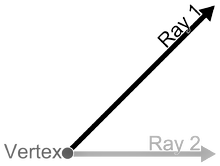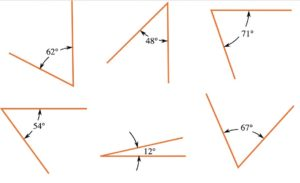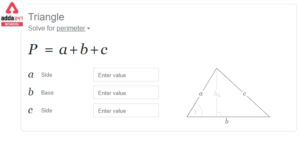Online Tution   »   Important Question   »   Acute angle

# Acute angle: Definition, Degree, Triangle, Example

## What is Acute angle?

An angle is a figure created by two rays, called the sides of the angle, that share a common endpoint, called the vertex of the angle, in Euclidean geometry. The plane that contains the rays contains the angles formed by two beams. Angles are also generated when two planes intersect. Dihedral angles are what they’re called. Two intersecting curves can also define an angle, which is the angle formed by rays lying tangent to the curves at their junction. Angle can also refer to the measurement of an angle or a rotation. The length of a circular arc divided by its radius is this measurement. The arc is centred at the vertex and defined by the sides in the case of a geometric angle. When a rotation occurs, the arc is centred at the rotation’s centre and delimited by any other point and the rotation’s image.Figure: Two rays forming an angle

## Acute Angle: Definition

Acute Angle Definition: An acute angle is one that is less than 90 degrees. The acute angle is also known as the angle that is smaller than 90 degrees. The acute angle is represented by the symbol and is measured in degrees using a protractor. Two lines intersect at a location to make an angle in mathematics. Acute, obtuse, right, and other forms of angles exist in geometry. An acute angle is one that is smaller than 90 degrees. Triangles, parallelograms, trapezoids, and other polygons all have at least one acute angle.Figure: Examples of Acute Angles

## Acute Angle: Triangle

Triangle: A triangle is a three-sided polygon with three vertices and three edges. It is a fundamental geometric shape. Triangle XYZ is a triangle with vertices X, Y, and Z. In Euclidean geometry, any three non-collinear points form a unique triangle and, at the same time, a unique plane.

Acute Angle Triangle: An acute-angled triangle is one in which all three internal angles of the triangle are acute, meaning they are less than 90 degrees. At the same time, a triangle cannot be sharp and right-angled. A triangle cannot be both obtuse and acute at the same time. There are three types of acute angle triangle.

### Acute Equilateral Triangle:

An equilateral acute triangle’s internal angles are all 60 degrees. An equi-angular triangle is another name for it.

### Acute Isosceles Triangle:

An isosceles acute triangle’s two angles, like its two sides, have the same measurement. E.g. Two angles of a triangle are equal to 55 degrees each and the remaining third angle is equal to 70 degrees.

### Acute Scalene Triangle:

A scalene acute triangle has three uneven sides and internal angles, all of which are less than 90 degrees. E.g. The three angles of a triangle are 50 degrees, 60 degrees and 70 degrees.

## Acute Angle and Obtuse Angle

The obtuse angle is defined as any angle that is more than 90 degrees. Angles range from 90 to 180 degrees. If we have the acute angle measurement, we can calculate an obtuse angle.

The obtuse Angle triangle is also the one in which in angle of the triangle is greater than 90 degrees. The other two angles of the triangle still remain less than 90 degrees or acute angles.

### Difference between Acute Angle and Obtuse Angles:

 Acute Angle Obtuse Angle Acute Angle is an Angle less than 90 degrees. An acute-angled triangle is one in which all three internal angles of the triangle are acute, meaning they are less than 90 degrees. Every Triangle at least has two acute angles even if it is an right triangle or obtuse angle triangle. Obtuse Angle is an angle grater than 90 degrees. The obtuse Angle triangle is also the one in which in angle of the triangle is greater than 90 degrees. There can only be one obtuse angle in a triangle. In right triangle and acute angled triangle, there are no obtuse angles.

Related Post:

## Acute Angle: FAQs

Ques. What is acute angle example?

Ans. Any angle that is less than 90 degrees in its measurement is an acute angle. Therefore, the examples of Acute angle are all angles between 0 degrees and 90 degress, i.e. 10 degrees, 20 degrees, 30 degrees, 45 degrees, etc.

Ques. What is an obtuse triangle called?

Ans. Obtuse-angled triangle or obtuse triangle is a triangle in which one of the angles is an obtuse angle or greater than 90 degrees. The inner angles of an obtuse triangle are all equal to 180 degrees.

Ques. What is reflex angle?

Ans. Any angle that is greater than 180 degrees (half circle) but less than 360 degrees is referred to as a reflex angle (full circle). On the other side of a reflex angle, there will always be an obtuse or acute angle.

Sharing is caring!

## FAQs

### What is acute angle example?

Any angle that is less than 90 degrees in its measurement is an acute angle. Therefore, the examples of Acute angle are all angles between 0 degrees and 90 degress, i.e. 10 degrees, 20 degrees, 30 degrees, 45 degrees, etc.

### What is an obtuse triangle called?

Obtuse-angled triangle or obtuse triangle is a triangle in which one of the angles is an obtuse angle or greater than 90 degrees. The inner angles of an obtuse triangle are all equal to 180 degrees.

### What is reflex angle?

Any angle that is greater than 180 degrees (half circle) but less than 360 degrees is referred to as a reflex angle (full circle). On the other side of a reflex angle, there will always be an obtuse or acute angle.

### TOPICS:

•Perimeter of Triangle- Formula, Definiti...